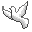Community Discussion list Contact Andrius Kulikauskas m a t h 4 w i s d o m @ g m a i l . c o m +370 607 27 665 Eičiūnų km, Alytaus raj, LithuaniaMy original work is in the Public Domain for you to use in your best judgement. Thank you for your support! Patreon Paypal to ms@ms.ltBookshelfAs an Amazon Associate I earn from qualifying purchases. Thank you! Upload Here is some text for a video that I am making about linear regression. Linear regression Linear regression. Do you have intuition to share about linear regression? Or would you like to learn along with me, as a seeker of wisdom? I asked John Harland to teach me the main idea of linear regression from his perspective. John and I met as PhD students in mathematics at the University of California at San Diego in 1986. He did his PhD in functional analysis and that informs his point of view. John teaches math at Palomar College in San Marcos, California, including several courses on statistics. He has a passion for the fundamentals of physics, and these last two years we have been talking regularly about that. He participates in the Math 4 Wisdom discussion group. His deepest value is "The experience of life" and he wonders "Is there a way of understanding how the laws of physics could have evolved from something simpler?" I am Andrius Kulikauskas. I host Math 4 Wisdom where I work with others to uncover a language of cognitive frameworks and express it in the language of advanced mathematics. I recently learned about the generalized linear model, which is a generalization of ordinary linear regression. The generalized linear model relates to the natural exponential families with quadratic variance function, which mirror the fivefold classification of the orthogonal Sheffer polynomials. I am making videos about my investigations of this fivefold classification and how it may express the fivesome, a cognitive framework for decision-making, whereby "every effect has had its cause; but not every cause has had its effects; so there is a critical point for deciding". In order to understand the generalized linear model, I should first understand linear regression. As a physics student, I learned the method of least squares, which is used to calculate a line that best fits a set of data points in an x-y plane, where we minimize the sum of the squares of the distances from the data points to the best fitting line, which is the regression line. I suppose that this is the heart of linear regression, although other norms may be used for best fit, not necessarily least squares. In tutoring me, John keeps returning to the question, on what basis can we say that least squares yields the best estimation? That's a question we don't answer in this video but hopefully will in a future video, perhaps with your help! I welcome your comments below and invite you to contact me. I recorded two sessions with John. I have reorganized the material so that you can start with the key ideas sooner. In this first excerpt, I'm trying to restate one of the main ideas that he taught me. John has written down a formula for the slope {$k$} of the regression line {$y=kx+b$}, which models the expected value of the conditional probability of {$y$} given {$x$}. According to this formula, as {$n$} goes to infinity, {$k$} approaches {$\sigma_{XY}/\sigma_x^2$}, which is the covariance over the square of the variance of {$x$}. Here is how I've organized the material. First, John will give the big picture of his understanding, that the real value of the regression line is the ability to predict how reliable it is. Next, I will share the insight that John inspired for me, which is to hypothesize an adjunction by which linear regression relates a world of datasets with a world of linear relations. Going back and forth between these worlds manifests four levels of knowledge: whether, what, how, why, where reliability is why we use linear regression. Then, we will proceed with the main part, where John explains to me linear regression from the point of view of a joint density function and estimating the terms in the covariance matrix. Finally, John and I have a discussion on cognitive frameworks (such as one, all, many), and on a game theory approach to making the best predictions, and the metalevels involved in such an analysis, and an example of how optimization and morality diverge. So let's start with the big picture!
Šis puslapis paskutinį kartą keistas December 22, 2022, at 07:50 PM